MachineMfg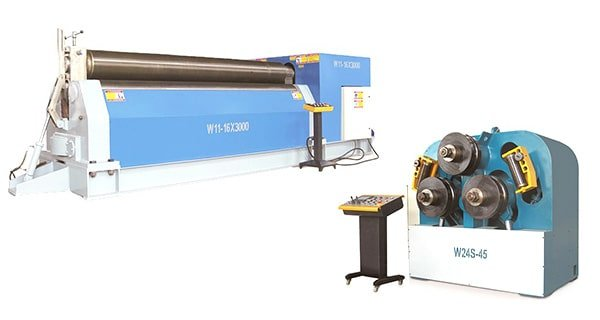## Rolling Machine

We provide you both the 3-roller plate rolls and 4-roller plate rolls, including mechanical type and hydraulic type. We also provide the profile bending machine to roll angle steel, H beam, I beam etc.

### Get A Quick Quote Now​

We will choose the most suitable press brake toolings for your product.

Click or drag a file to this area to upload.

# Load Analysis and Driven Power Calculation (Symmetrical 3 Roll Plate Bending Machine)

## Introduction

Since the load on the plate roll bending machine is large, so the requirement for the parts strength is high.

In addition, due to the fierce competition in the market, it is urgent to reduce the cost of the plate rolls, so it is imperative to design the machine accurately and reliably.

For the design calculation of the roll bending machine, the force analysis of the rolling machine is required first, and the result is the original parameters of the design of each part of the rolling machine.

And the calculation of driving power of the main drive system is the necessary parameter for designing the main drive system and selecting the motor.

Therefore, the calculation of the force analysis and driving power of the plate rolling machine is very important to the design of the roll bending machine.

In this post, the calculation of the force capabilities of symmetrical three-roll bending machine is one of the methods, the other types of plate rolling machine can take it for reference.

## Force Analysis

### 2.1 Maximum torque required for a cylinder rolling

When the plate rolling machine is working, the steel sheet should be rolled into the steel pipe. At this time, the stress of the material has reached the yield limit.

Therefore, the bending stress distribution on the tube section is shown below the figure (b), and the bending moment M of the section is: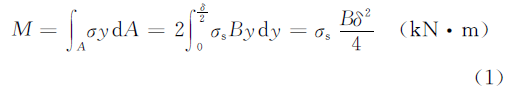In the above formula,

B, δ – The maximum width and thickness of rolled steel sheet (m)

`σs - Material's yield limit (kN • m-2)`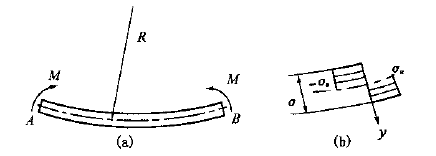Fig.1 Stress distribution of roll bending

When considering the deformation of the material, there is reinforcement, and the reinforcement coefficient K is introduced to modify the equation (1), namely: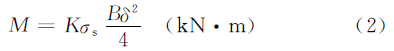In the above formula,

K – reinforcement coefficient, the value can be K = 1.10~1.25, when the result for δ/R is big, then take the biggest value.

R – Neutral layer’s radius of the rolling plate (m)

### 2.2 Force Condition

When rolling steel plate, the force condition is shown as below figure. According to the force balance, the supporting force Fon the roll plate can be obtained via the formula: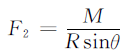In the above formula,

θ – The angle between defiled line OO1 and OO2,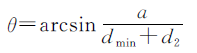α – Lower roller center distance (m)

dmin – Min diameter of plate rolling (m)

d2Lower roller diameter (m)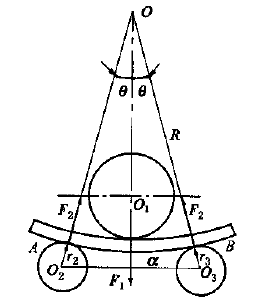Fig.2 Force analysis of roll bending

Considering that the thickness of the plate δ is far less than the minimum diameter of the rolling tube, the radius R of the neutral layer is around 0.5dmin, in order to simplify the calculation , the above equation can be changed to: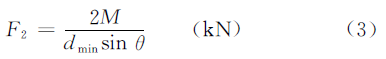According to the force balance, the pressure force F1, which is generated by the upper roller, acting on the rolling plate is: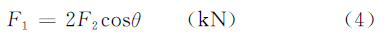## Calculation of driving power

### 3.1 Lower roller drive moment

The lower roller of the plate rolling machine is the driving roller, and the driving torque on the lower roller is used to overcome the deformation torque Tn1 and the friction torque Tn2.

In the process of steel plate rolling, the deformation capabilities which stored in AB section of steel plate (see Fig 1a and Fig 2) is 2Mθ, the costed time is 2θR/V (V is rolling speed).

The ratio is equal to the power of deformation torque Tn1, namely:```Therefore,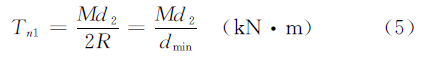```

The friction torque includes the rolling friction torque between the upper and lower roller and the steel plate, and the sliding friction torque between the roller neck and the shaft sleeve, which can be calculated as follows:In the above formula:

f – Coefficient of rolling friction, take f = 0.008m

μ – Coefficient of sliding friction, take μ = 0.05-0.1d1,

d2 – Upper roller & lower roller diameter (m)

D1, D2 – Upper roller & lower roller neck diameter (m)

The size is not yet accurate in the design phase, the value can take Di = 0.5di (i=1, 2). The lower roller drive torque T equals the sum of the deformation torque Tn1 and the friction torque Tn2.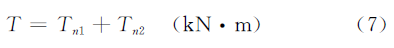### 3.2 Lower roller driven power

Lower roller driven power is:In the above formula:

P – Driven power (m • KW)

T – Driven force moment (KN • m)

n2 – Lower roller rotation speed (r • min-1), n2=2V/d2 (V is rolling speed)

η – transmission efficiency， η=0.65-0.8

The power of the main motor can be obtained from the value of P.

## Sharing is Awesome, Do It!

### 29 thoughts on “Load Analysis and Driven Power Calculation (Symmetrical 3 Roll Plate Bending Machine)”

1. Sir,
I made a 3 roller machine of bed length 3600mm, But when tried for bending the center part of the sheet did not bend . The diameter of 3 shafts is 58mm. Thicknes of the sheet is1.6mm., Kindly guide me through this issue

2. dear sir,
I want to know that what is the distance between two rollers of sheet rolling machine. because I am traying to make one sheet rolling machine. so please guide me the distance between two rollers( bottom) . my roller dia is 120mm all three rollers.

3. Sir, how we can decide the roller diameter if we want to make the pipe of 25 to 50 mm dia.from 1.5- 2 mm sheet thickness,and also please tell us about other design calculations like speed of roller, bottom roller distance etc..

To Get Our Lastest Articles!
Subscribe
Your information will never be shared